# ML Aggarwal Solutions for Class 6 Maths Chapter 11 Understanding Symmetrical Shapes

ML Aggarwal Solutions for Class 6 Maths Chapter 11 Understanding Symmetrical Shapes are available in PDF format. ML Aggarwal problems assist students in developing problem-solving and higher-order thinking skills. It aims to provide students with a variety of quality problems in different formats. Students who wish to score good marks in Maths, practice ML Aggarwal Solutions for Class 6 Maths Chapter 11.

Chapter 11 – Understanding Symmetrical Shapes consists of problems based on the concept of symmetrical shapes. The images which are divided into identical halves are known as symmetrical. A shape has symmetry if a central dividing line can be drawn on it. This Chapter contains different concepts of symmetrical shapes and also students learn the use of divider and ruler to measure the length.

## Download the PDF of ML Aggarwal Solutions for Class 6 Maths Chapter 11 Understanding Symmetrical Shapes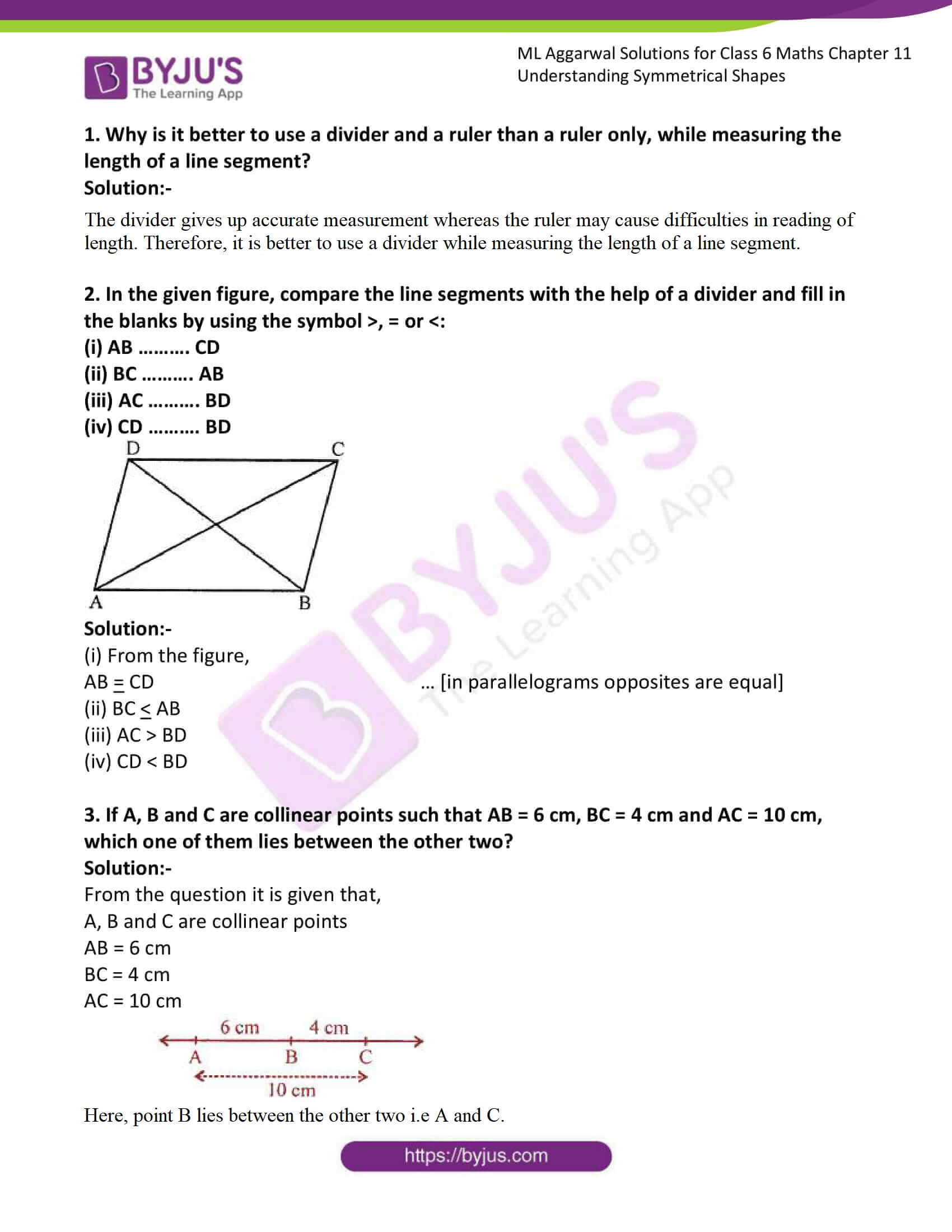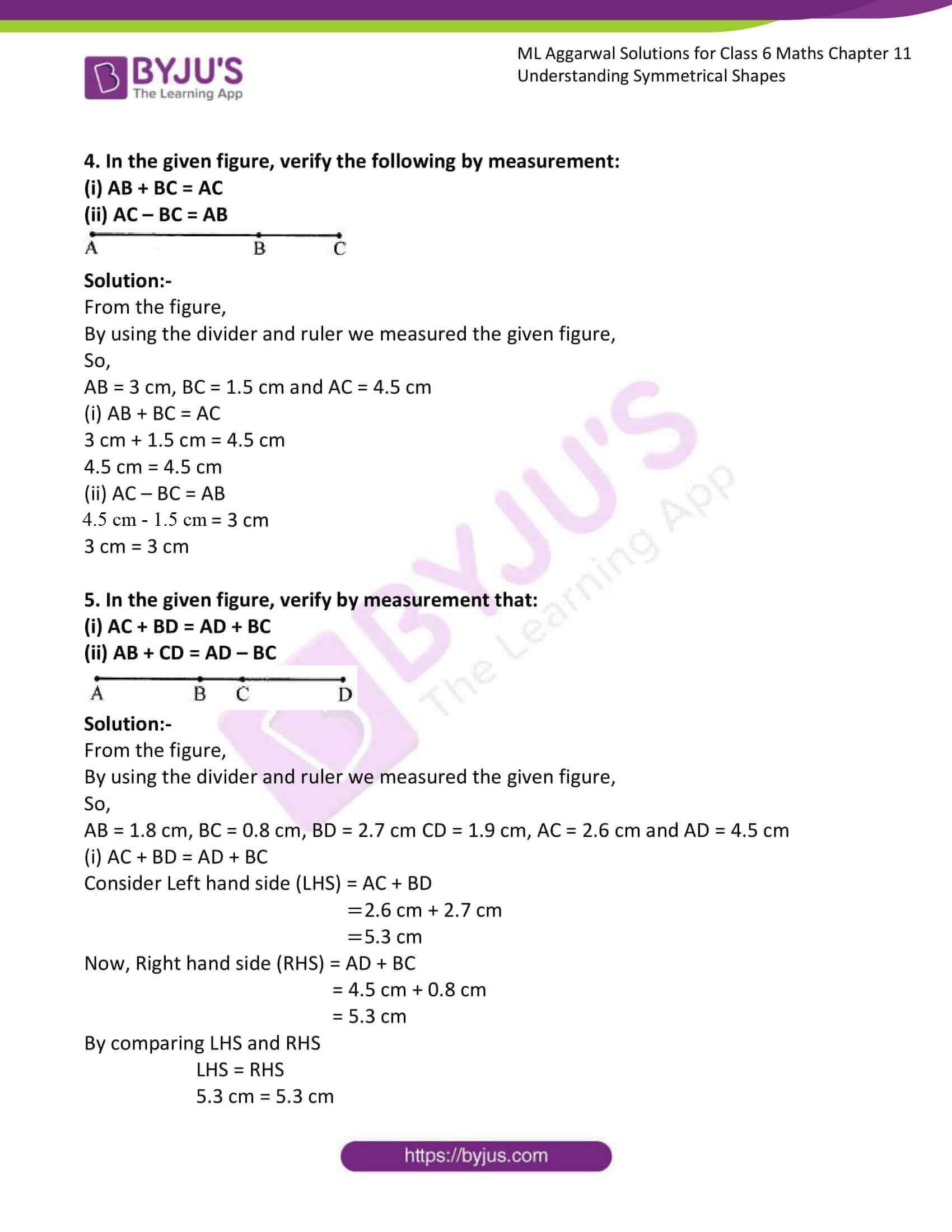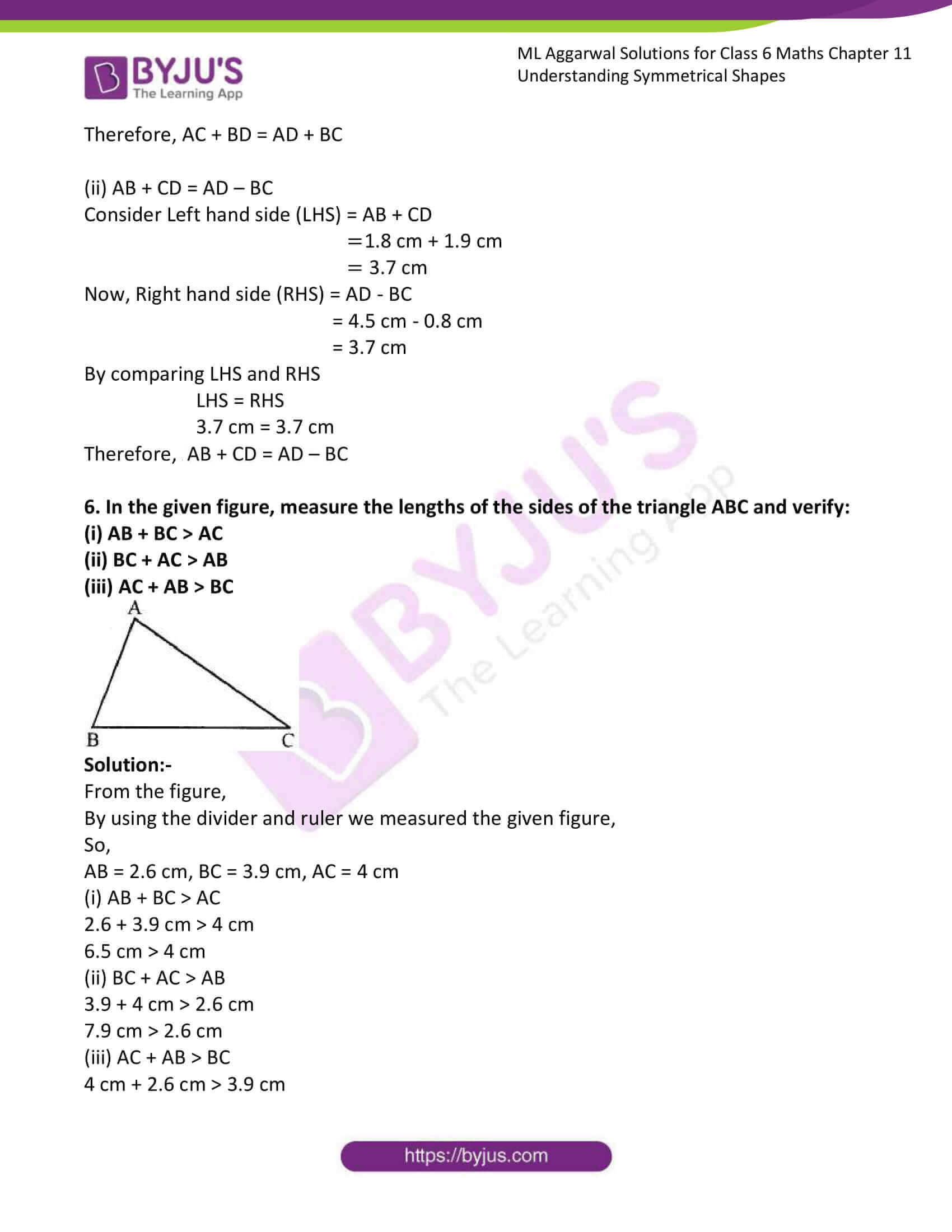### Access answers to ML Aggarwal Solutions for Class 6 Maths Chapter 11 Understanding Symmetrical Shapes

1. Why is it better to use a divider and a ruler than a ruler only, while measuring the length of a line segment?
Solution:-

The divider gives up accurate measurement whereas the ruler may cause difficulties in reading of length. Therefore, it is better to use a divider while measuring the length of a line segment.

2. In the given figure, compare the line segments with the help of a divider and fill in the blanks by using the symbol >, = or <:
(i) AB ………. CD
(ii) BC ………. AB
(iii) AC ………. BD
(iv) CD ………. BD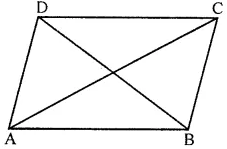Solution:-

(i) From the figure,

AB = CD … [in parallelograms opposites are equal]

(ii) BC < AB

(iii) AC > BD

(iv) CD < BD

3. If A, B and C are collinear points such that AB = 6 cm, BC = 4 cm and AC = 10 cm, which one of them lies between the other two?
Solution:-

From the question it is given that,

A, B and C are collinear points

AB = 6 cm

BC = 4 cm

AC = 10 cm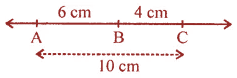Here, point B lies between the other two i.e A and C.

4. In the given figure, verify the following by measurement:
(i) AB + BC = AC
(ii) AC – BC = ABSolution:-

From the figure,

By using the divider and ruler we measured the given figure,

So,

AB = 3 cm, BC = 1.5 cm and AC = 4.5 cm

(i) AB + BC = AC

3 cm + 1.5 cm = 4.5 cm

4.5 cm = 4.5 cm

(ii) AC – BC = AB

4.5 cm – 1.5 cm = 3 cm

3 cm = 3 cm

5. In the given figure, verify by measurement that:
(i) AC + BD = AD + BC
(ii) AB + CD = AD – BC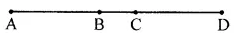Solution:-

From the figure,

By using the divider and ruler we measured the given figure,

So,

AB = 1.8 cm, BC = 0.8 cm, BD = 2.7 cm CD = 1.9 cm, AC = 2.6 cm and AD = 4.5 cm

(i) AC + BD = AD + BC

Consider Left hand side (LHS) = AC + BD

= 2.6 cm + 2.7 cm

= 5.3 cm

Now, Right hand side (RHS) = AD + BC

= 4.5 cm + 0.8 cm

= 5.3 cm

By comparing LHS and RHS

LHS = RHS

5.3 cm = 5.3 cm

Therefore, AC + BD = AD + BC

(ii) AB + CD = AD – BC

Consider Left hand side (LHS) = AB + CD

= 1.8 cm + 1.9 cm

= 3.7 cm

Now, Right hand side (RHS) = AD – BC

= 4.5 cm – 0.8 cm

= 3.7 cm

By comparing LHS and RHS

LHS = RHS

3.7 cm = 3.7 cm

Therefore, AB + CD = AD – BC

6. In the given figure, measure the lengths of the sides of the triangle ABC and verify:
(i) AB + BC > AC
(ii) BC + AC > AB
(iii) AC + AB > BC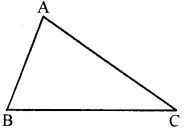Solution:-

From the figure,

By using the divider and ruler we measured the given figure,

So,

AB = 2.6 cm, BC = 3.9 cm, AC = 4 cm

(i) AB + BC > AC

2.6 + 3.9 cm > 4 cm

6.5 cm > 4 cm

(ii) BC + AC > AB

3.9 + 4 cm > 2.6 cm

7.9 cm > 2.6 cm

(iii) AC + AB > BC

4 cm + 2.6 cm > 3.9 cm

6.6 cm > 3.9 cm